# RS Aggarwal Solutions for Class 7 Maths Exercise 7B Chapter 7 Linear Equations in One Variable

RS Aggarwal Solutions for Class 7 Maths Exercise 7B Chapter 7 Linear Equations in One Variable, Maths is a subject which requires understanding and reasoning skills accompanied by logic. Along with this, it also requires students to practice Maths regularly. This exercise contains problems based on linear equations. Students of Class 7 are suggested to solve RS Aggarwal Class 7 Solutions Chapter 7 to understand and crack these problems. We at BYJU’S have prepared the RS Aggarwal Solutions for Class 7 Maths Chapter 7 Linear Equations in One Variable wherein problems are solved step by step with in-depth explanations.

## Download the PDF of RS Aggarwal Solutions For Class 7 Maths Chapter 7 Linear Equations in One Variable – Exercise 7B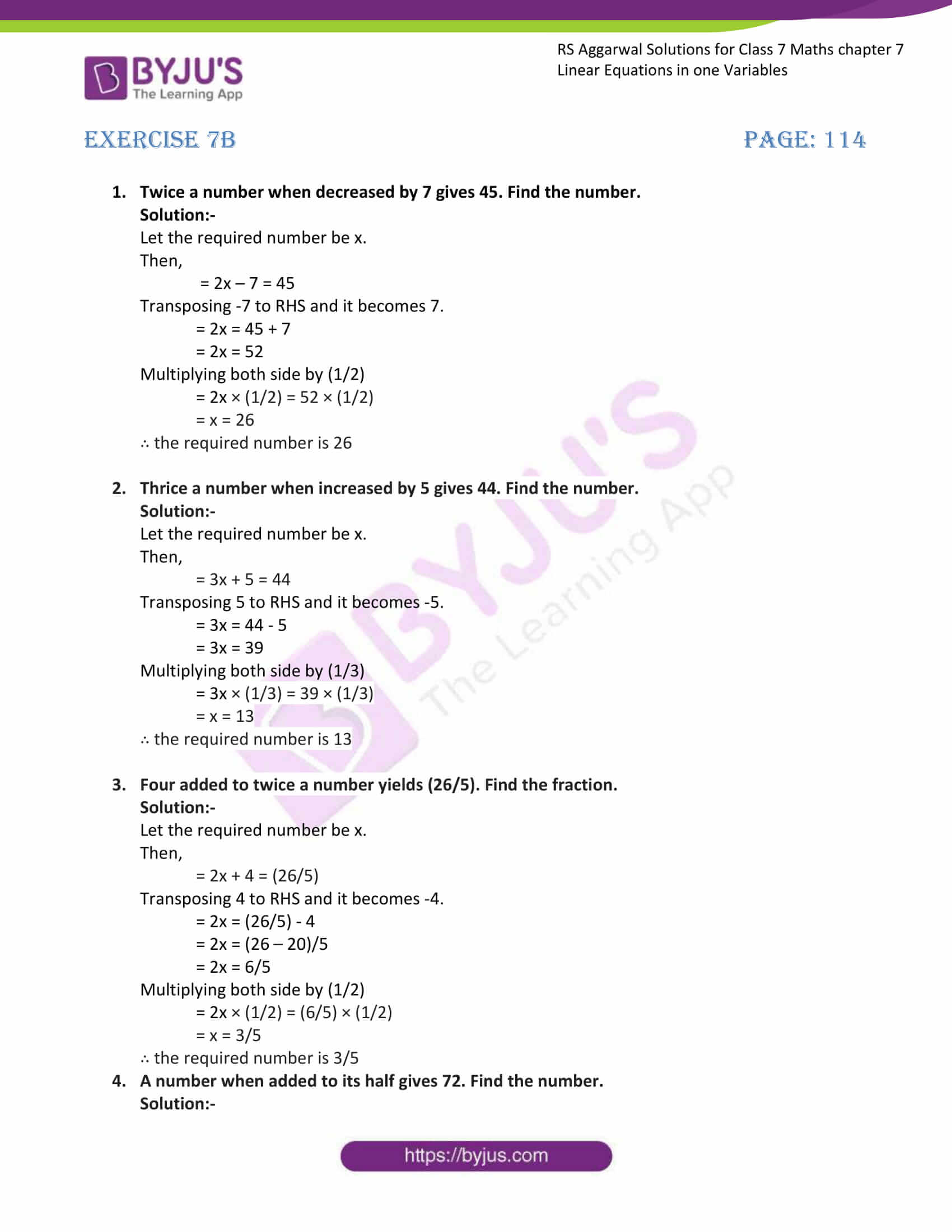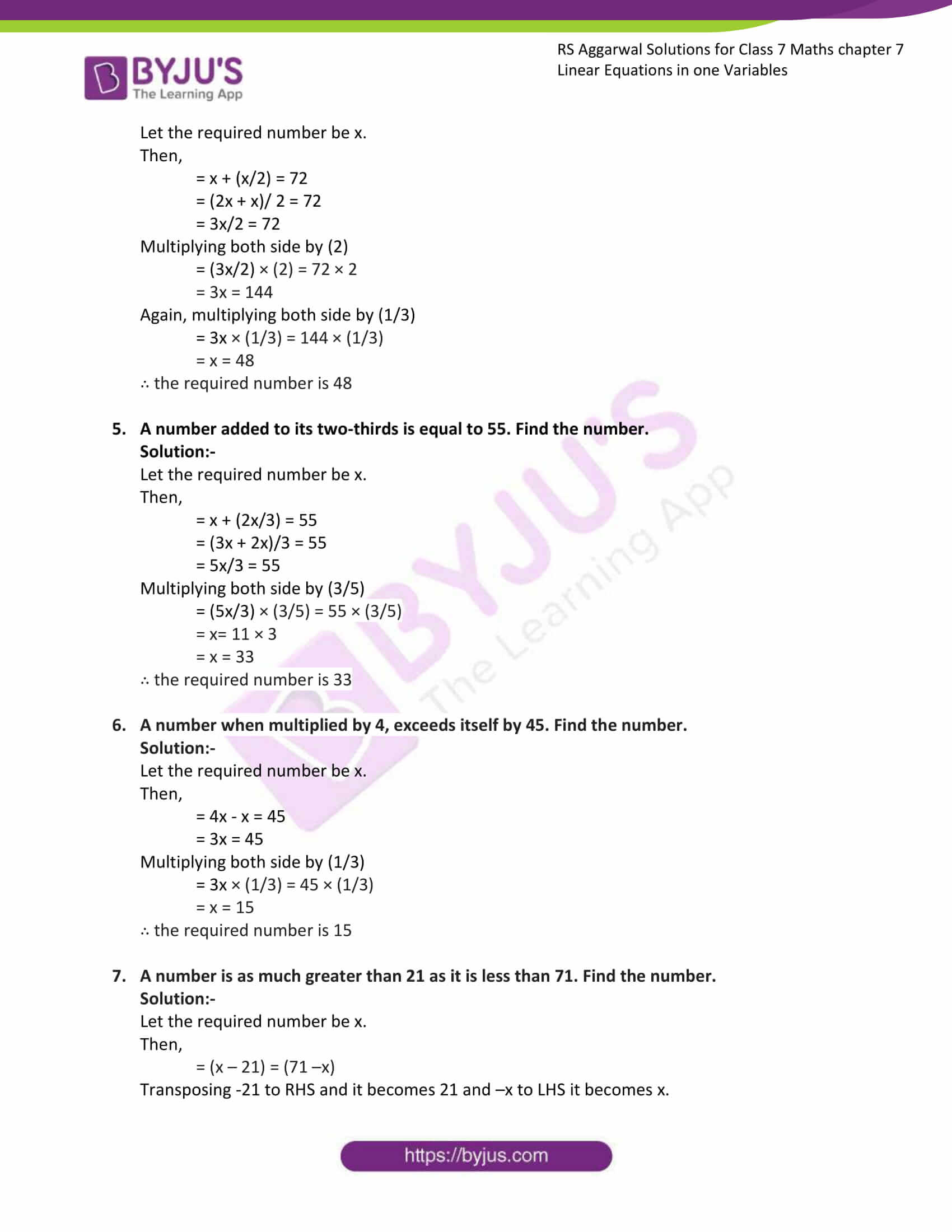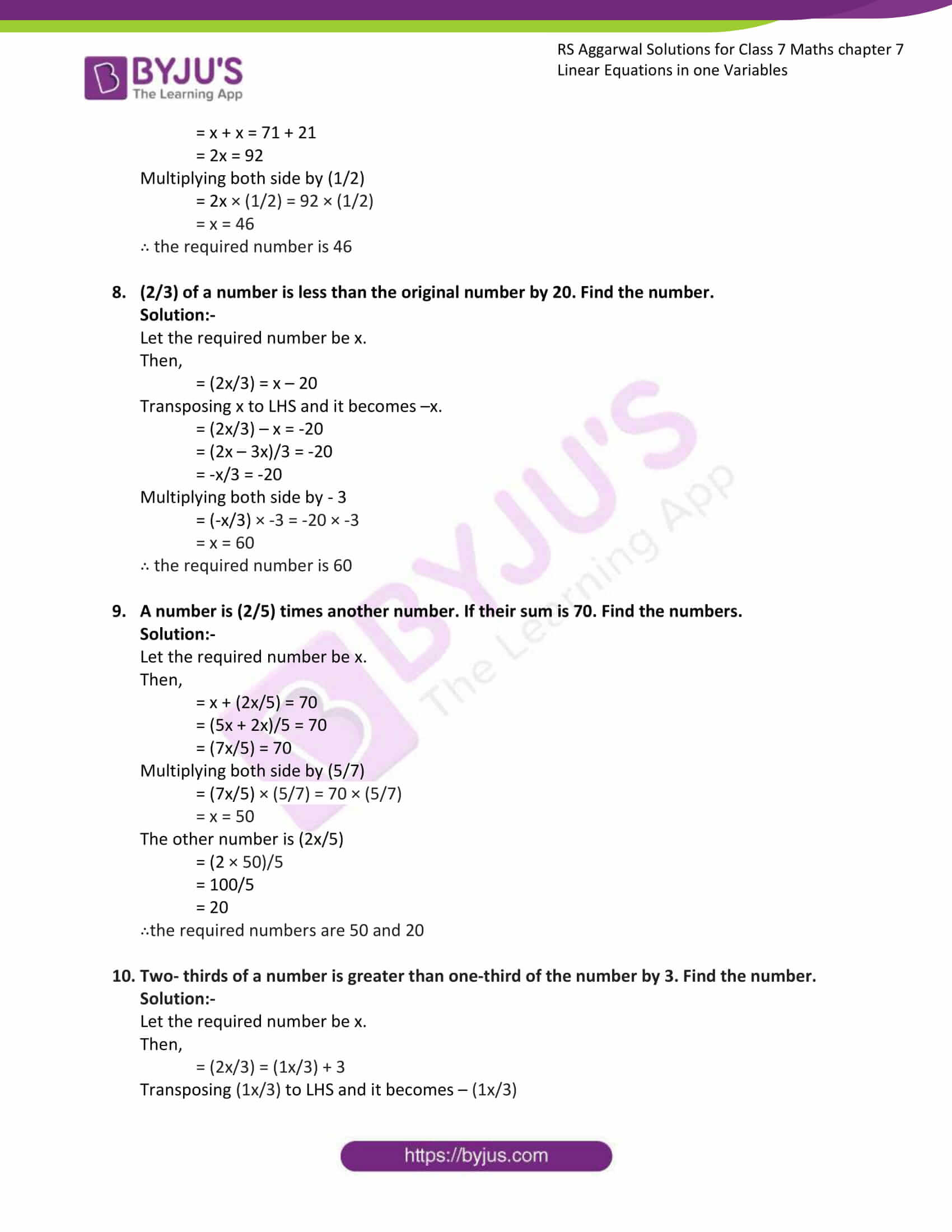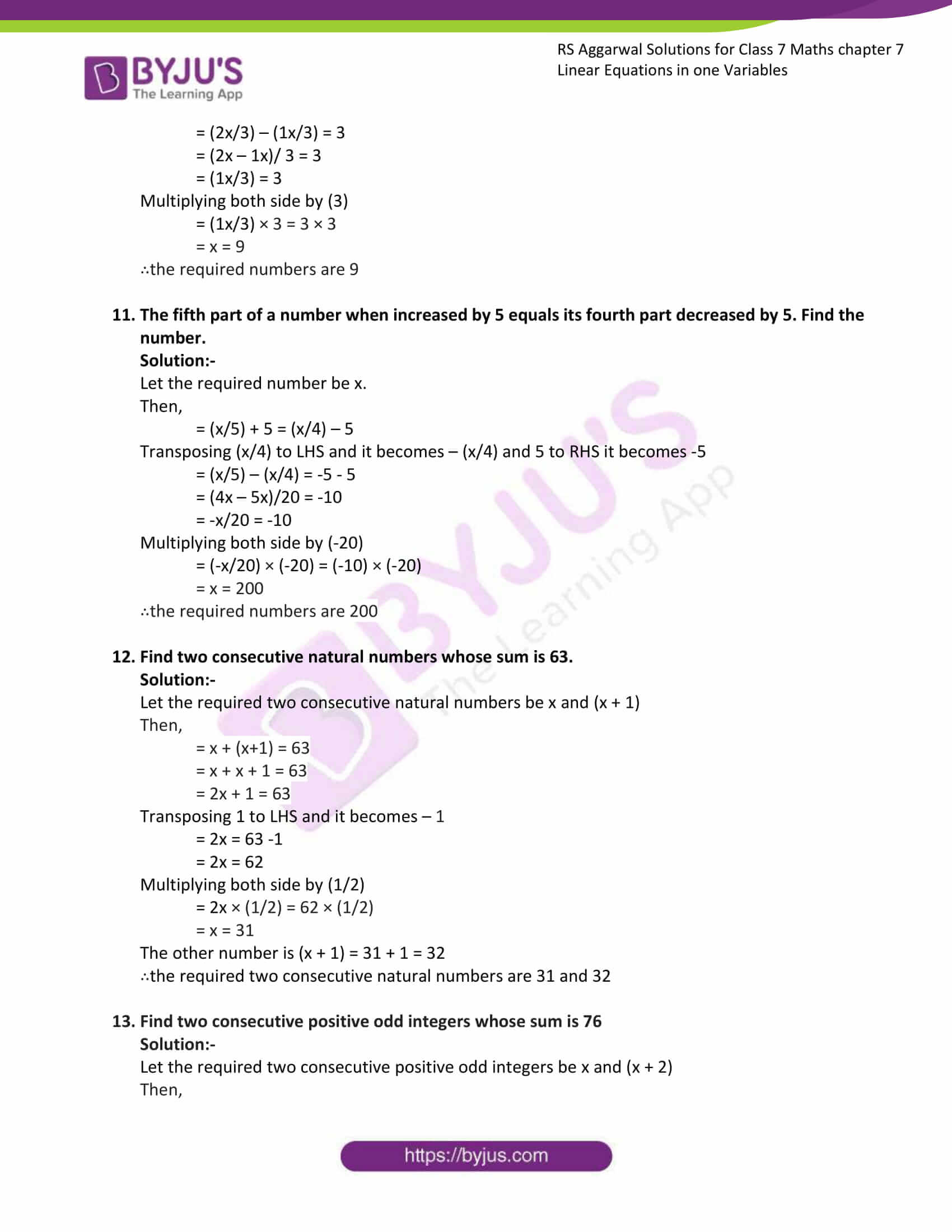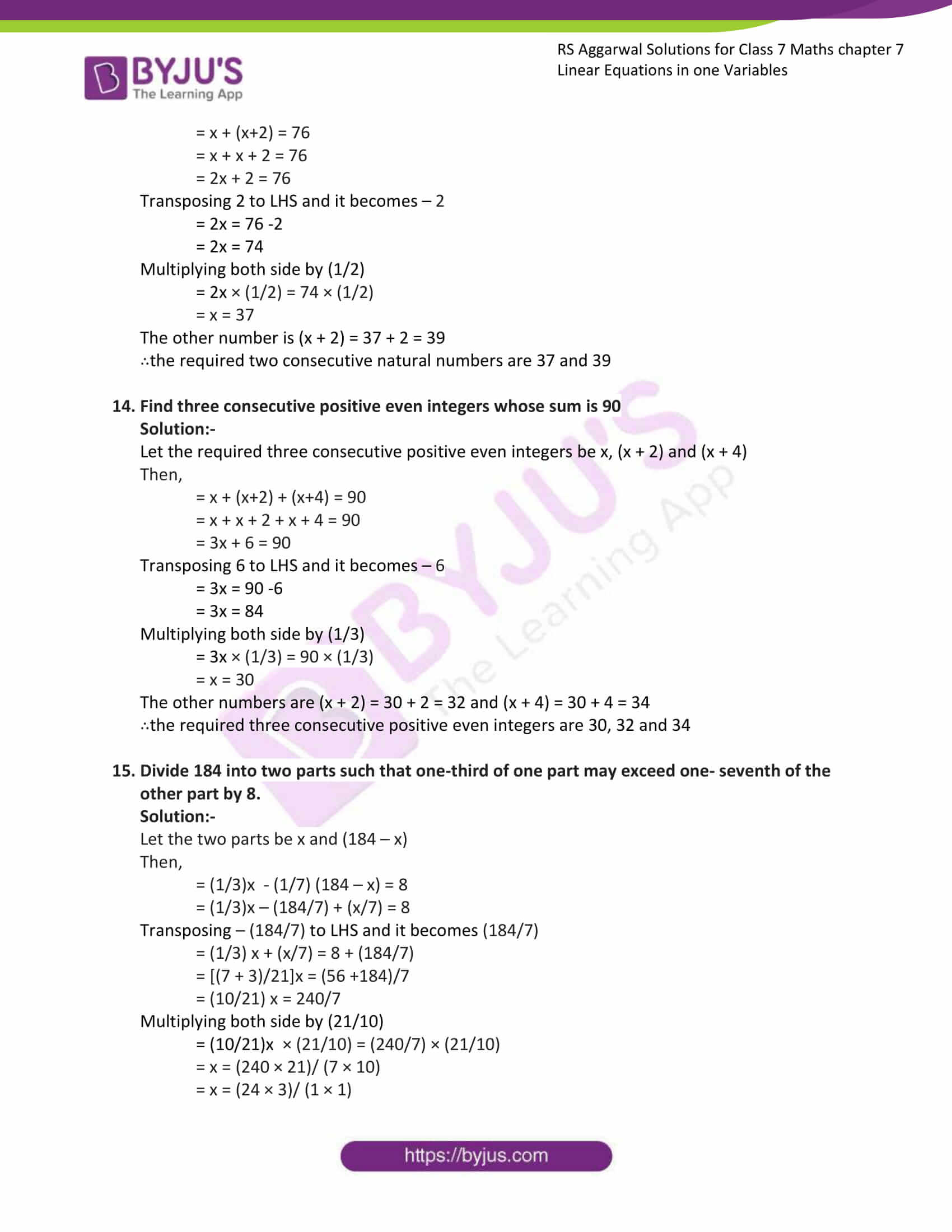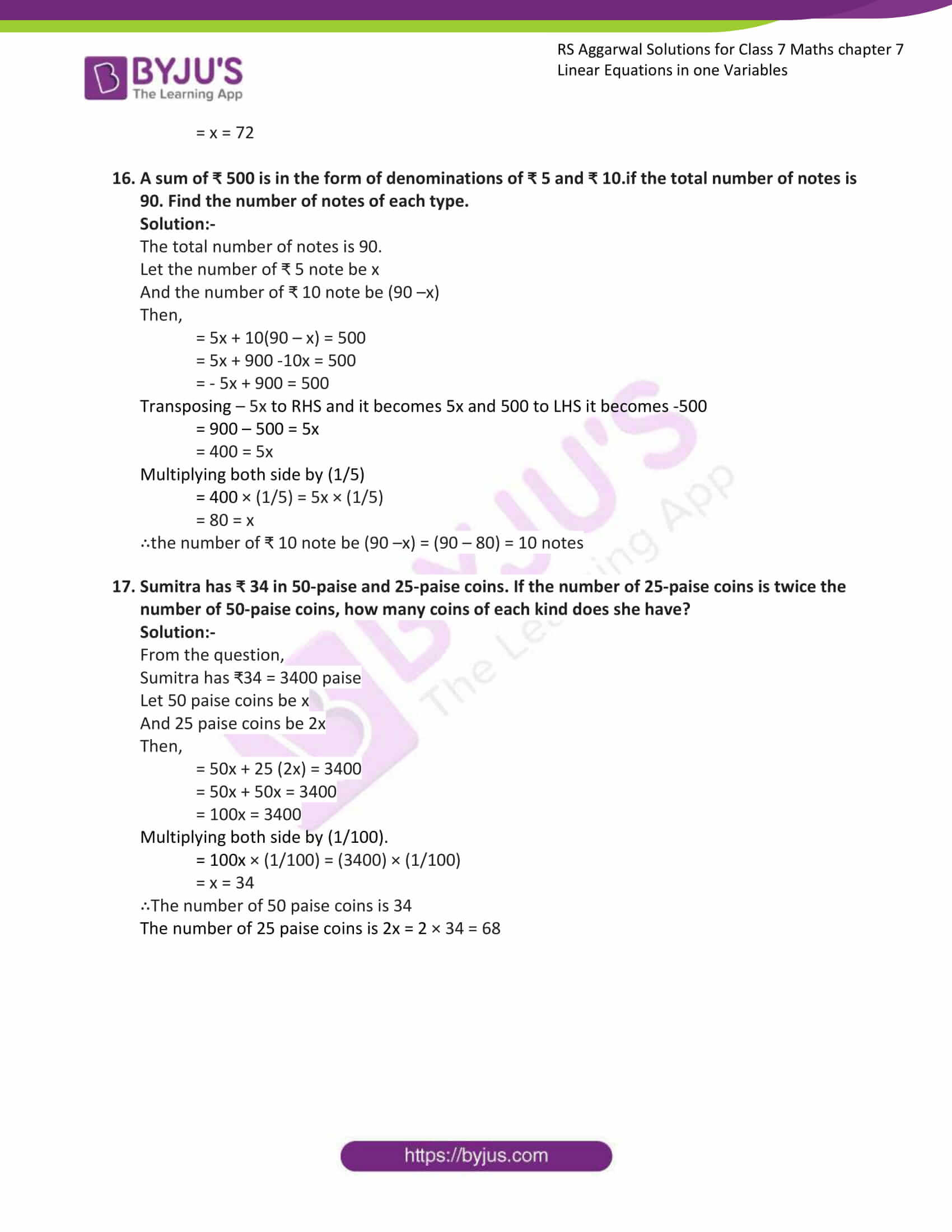### Access answers to Maths RS Aggarwal Solutions for Class 7 Chapter 7 – Linear Equations in One Variable Exercise 7B

1. Twice a number when decreased by 7 gives 45. Find the number.

Solution:-

Let the required number be x.

Then,

= 2x – 7 = 45

Transposing -7 to RHS and it becomes 7.

= 2x = 45 + 7

= 2x = 52

Multiplying both side by (1/2)

= 2x × (1/2) = 52 × (1/2)

= x = 26

∴ the required number is 26

2. Thrice a number when increased by 5 gives 44. Find the number.

Solution:-

Let the required number be x.

Then,

= 3x + 5 = 44

Transposing 5 to RHS and it becomes -5.

= 3x = 44 – 5

= 3x = 39

Multiplying both side by (1/3)

= 3x × (1/3) = 39 × (1/3)

= x = 13

∴ the required number is 13

3. Four added to twice a number yields (26/5). Find the fraction.

Solution:-

Let the required number be x.

Then,

= 2x + 4 = (26/5)

Transposing 4 to RHS and it becomes -4.

= 2x = (26/5) – 4

= 2x = (26 – 20)/5

= 2x = 6/5

Multiplying both side by (1/2)

= 2x × (1/2) = (6/5) × (1/2)

= x = 3/5

∴ the required number is 3/5

4. A number when added to its half gives 72. Find the number.

Solution:-

Let the required number be x.

Then,

= x + (x/2) = 72

= (2x + x)/ 2 = 72

= 3x/2 = 72

Multiplying both side by (2)

= (3x/2) × (2) = 72 × 2

= 3x = 144

Again, multiplying both side by (1/3)

= 3x × (1/3) = 144 × (1/3)

= x = 48

∴ the required number is 48

5. A number added to its two-thirds is equal to 55. Find the number.

Solution:-

Let the required number be x.

Then,

= x + (2x/3) = 55

= (3x + 2x)/3 = 55

= 5x/3 = 55

Multiplying both side by (3/5)

= (5x/3) × (3/5) = 55 × (3/5)

= x= 11 × 3

= x = 33

∴ the required number is 33

6. A number when multiplied by 4, exceeds itself by 45. Find the number.

Solution:-

Let the required number be x.

Then,

= 4x – x = 45

= 3x = 45

Multiplying both side by (1/3)

= 3x × (1/3) = 45 × (1/3)

= x = 15

∴ the required number is 15

7. A number is as much greater than 21 as it is less than 71. Find the number.

Solution:-

Let the required number be x.

Then,

= (x – 21) = (71 –x)

Transposing -21 to RHS and it becomes 21 and –x to LHS it becomes x.

= x + x = 71 + 21

= 2x = 92

Multiplying both side by (1/2)

= 2x × (1/2) = 92 × (1/2)

= x = 46

∴ the required number is 46

8. (2/3) of a number is less than the original number by 20. Find the number.

Solution:-

Let the required number be x.

Then,

= (2x/3) = x – 20

Transposing x to LHS and it becomes –x.

= (2x/3) – x = -20

= (2x – 3x)/3 = -20

= -x/3 = -20

Multiplying both side by – 3

= (-x/3) × -3 = -20 × -3

= x = 60

∴ the required number is 60

9. A number is (2/5) times another number. If their sum is 70. Find the numbers.

Solution:-

Let the required number be x.

Then,

= x + (2x/5) = 70

= (5x + 2x)/5 = 70

= (7x/5) = 70

Multiplying both side by (5/7)

= (7x/5) × (5/7) = 70 × (5/7)

= x = 50

The other number is (2x/5)

= (2 × 50)/5

= 100/5

= 20

∴the required numbers are 50 and 20

10. Two- thirds of a number is greater than one-third of the number by 3. Find the number.

Solution:-

Let the required number be x.

Then,

= (2x/3) = (1x/3) + 3

Transposing (1x/3) to LHS and it becomes – (1x/3)

= (2x/3) – (1x/3) = 3

= (2x – 1x)/ 3 = 3

= (1x/3) = 3

Multiplying both side by (3)

= (1x/3) × 3 = 3 × 3

= x = 9

∴the required numbers are 9

11. The fifth part of a number when increased by 5 equals its fourth part decreased by 5. Find the number.

Solution:-

Let the required number be x.

Then,

= (x/5) + 5 = (x/4) – 5

Transposing (x/4) to LHS and it becomes – (x/4) and 5 to RHS it becomes -5

= (x/5) – (x/4) = -5 – 5

= (4x – 5x)/20 = -10

= -x/20 = -10

Multiplying both side by (-20)

= (-x/20) × (-20) = (-10) × (-20)

= x = 200

∴the required numbers are 200

12. Find two consecutive natural numbers whose sum is 63.

Solution:-

Let the required two consecutive natural numbers be x and (x + 1)

Then,

= x + (x+1) = 63

= x + x + 1 = 63

= 2x + 1 = 63

Transposing 1 to LHS and it becomes – 1

= 2x = 63 -1

= 2x = 62

Multiplying both side by (1/2)

= 2x × (1/2) = 62 × (1/2)

= x = 31

The other number is (x + 1) = 31 + 1 = 32

∴the required two consecutive natural numbers are 31 and 32

13. Find two consecutive positive odd integers whose sum is 76

Solution:-

Let the required two consecutive positive odd integers be x and (x + 2)

Then,

= x + (x+2) = 76

= x + x + 2 = 76

= 2x + 2 = 76

Transposing 2 to LHS and it becomes – 2

= 2x = 76 -2

= 2x = 74

Multiplying both side by (1/2)

= 2x × (1/2) = 74 × (1/2)

= x = 37

The other number is (x + 2) = 37 + 2 = 39

∴the required two consecutive natural numbers are 37 and 39

14. Find three consecutive positive even integers whose sum is 90

Solution:-

Let the required three consecutive positive even integers be x, (x + 2) and (x + 4)

Then,

= x + (x+2) + (x+4) = 90

= x + x + 2 + x + 4 = 90

= 3x + 6 = 90

Transposing 6 to LHS and it becomes – 6

= 3x = 90 -6

= 3x = 84

Multiplying both side by (1/3)

= 3x × (1/3) = 90 × (1/3)

= x = 30

The other numbers are (x + 2) = 30 + 2 = 32 and (x + 4) = 30 + 4 = 34

∴the required three consecutive positive even integers are 30, 32 and 34

15. Divide 184 into two parts such that one-third of one part may exceed one- seventh of the other part by 8.

Solution:-

Let the two parts be x and (184 – x)

Then,

= (1/3)x – (1/7) (184 – x) = 8

= (1/3)x – (184/7) + (x/7) = 8

Transposing – (184/7) to LHS and it becomes (184/7)

= (1/3) x + (x/7) = 8 + (184/7)

= [(7 + 3)/21]x = (56 +184)/7

= (10/21) x = 240/7

Multiplying both side by (21/10)

= (10/21)x × (21/10) = (240/7) × (21/10)

= x = (240 × 21)/ (7 × 10)

= x = (24 × 3)/ (1 × 1)

= x = 72

16. A sum of ₹ 500 is in the form of denominations of ₹ 5 and ₹ 10.if the total number of notes is 90. Find the number of notes of each type.

Solution:-

The total number of notes is 90.

Let the number of ₹ 5 note be x

And the number of ₹ 10 note be (90 –x)

Then,

= 5x + 10(90 – x) = 500

= 5x + 900 -10x = 500

= – 5x + 900 = 500

Transposing – 5x to RHS and it becomes 5x and 500 to LHS it becomes -500

= 900 – 500 = 5x

= 400 = 5x

Multiplying both side by (1/5)

= 400 × (1/5) = 5x × (1/5)

= 80 = x

∴the number of ₹ 10 note be (90 –x) = (90 – 80) = 10 notes

17. Sumitra has ₹ 34 in 50-paise and 25-paise coins. If the number of 25-paise coins is twice the number of 50-paise coins, how many coins of each kind does she have?

Solution:-

From the question,

Sumitra has ₹34 = 3400 paise

Let 50 paise coins be x

And 25 paise coins be 2x

Then,

= 50x + 25 (2x) = 3400

= 50x + 50x = 3400

= 100x = 3400

Multiplying both side by (1/100).

= 100x × (1/100) = (3400) × (1/100)

= x = 34

∴The number of 50 paise coins is 34

The number of 25 paise coins is 2x = 2 × 34 = 68

### Access other exercises of RS Aggarwal Solutions For Class 7 Chapter 7 – Linear Equations in One Variable

Exercise 7C Solutions